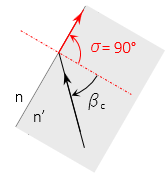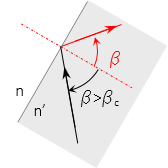## 4. Prisms

A prism is generally a glass block of a specific geometric shape, Figure H-7.(a) Right angle (b) Penta (c) Dove Figure H-7Common Prisms

Prisms are used to redirect and/or reorient light rays by refraction and reflection.

For light passing from a dense medium to a less dense one, Figure H-8, there is a critical incidence angle at which the refracted angle is exactly 90°Figure H-8Perpendicular Refraction

The light ray does not pass out of the denser medium. The incidence angle at which this occurs is the critical angle and can be computed using Equation H-3.Equation H-3

When the incidence angle exceeds the critical angle, the light ray will be entirely reflected, with no part of it refracting through the interface, Figure H-9.Figure H-9Internal Reflection

Prisms use this internal reflection to change the direction of light rays. Some prism applications in surveying that we'll cover later are:

• An optical plummet bends the line of sight 90° using a right angle prism
• A reflector for distance measurement uses three coinicident right angle prisms to reflect an electromagnetic signal back along its path
• An automatic level's compensator uses prisms to maintain a horizontal line of sight